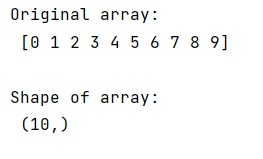# What does numpy ndarray shape do?

Learn about the numpy ndarray shape method with its examples.
Submitted by Pranit Sharma, on February 14, 2023

NumPy is an abbreviated form of Numerical Python. It is used for different types of scientific operations in python. Numpy is a vast library in python which is used for almost every kind of scientific or mathematical operation. It is itself an array which is a collection of various methods and functions for processing the arrays.

## numpy.ndarray.shape() Method

The numpy.ndarray.shape() returns the shape of our ndarray as a tuple. For a 1D array, the shape would be (n,) where n is the number of elements in your array.

For a 2D array, the shape would be (n,m) where n is the number of rows and m is the number of columns in your array.

The simple logic behind the output of .shape() is as follows:

• For 1D array, return a shape tuple with only 1 element (i.e. (n,))
• For 2D array, return a shape tuple with only 2 elements (i.e. (n,m))
• For 3D array, return a shape tuple with only 3 elements (i.e. (n,m,k))
• For 4D array, return a shape tuple with only 4 elements (i.e. (n,m,k,j))
• and so on.

Let us understand with the help of an example,

## Python code to demonstrate the example of numpy.ndarray.shape() method

```# Import numpy
import numpy as np

# Creating a numpy array
arr = np.arange(10)

# Display original array
print("Original array:\n",arr,"\n")

# Display shape
print("Shape of array:\n",np.shape(arr))
```

Output: